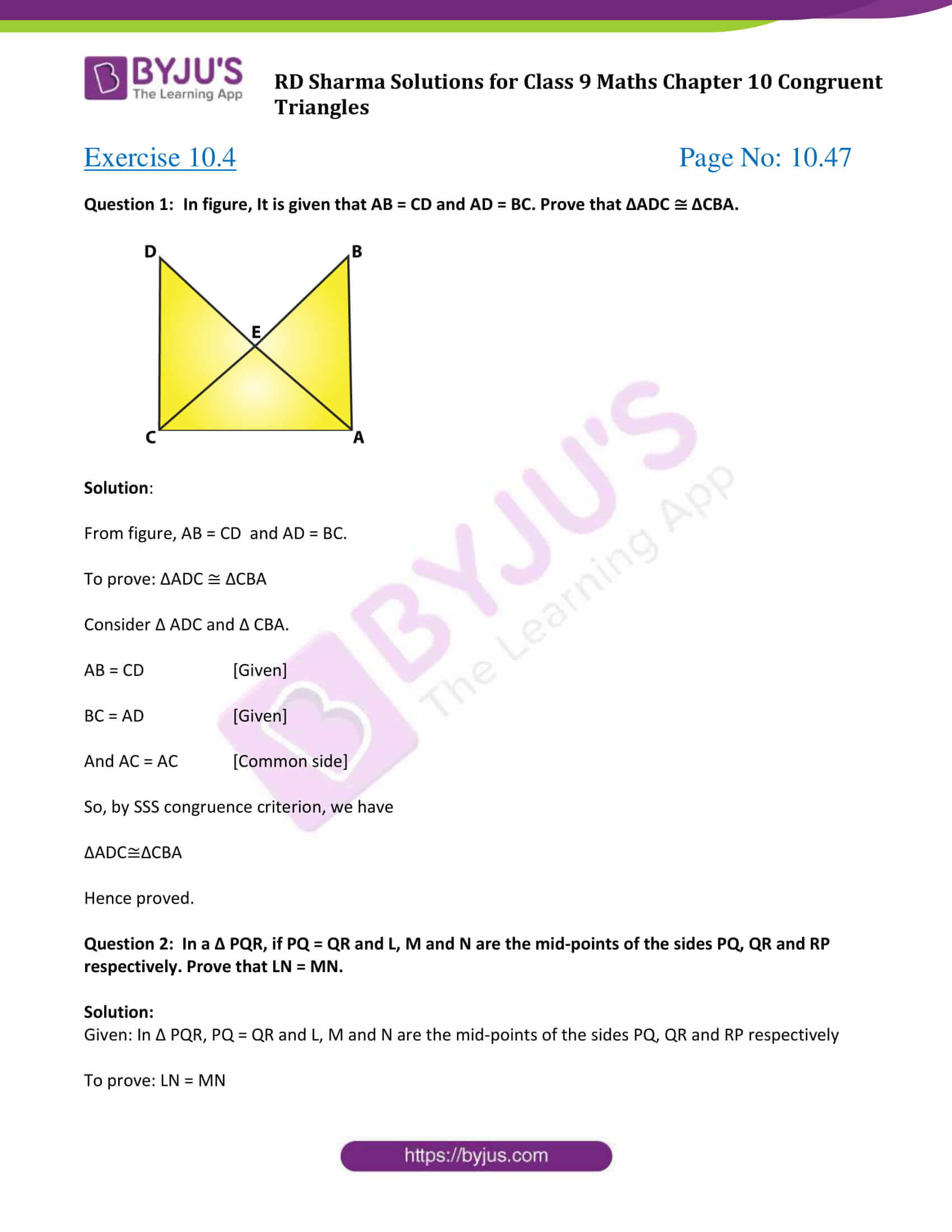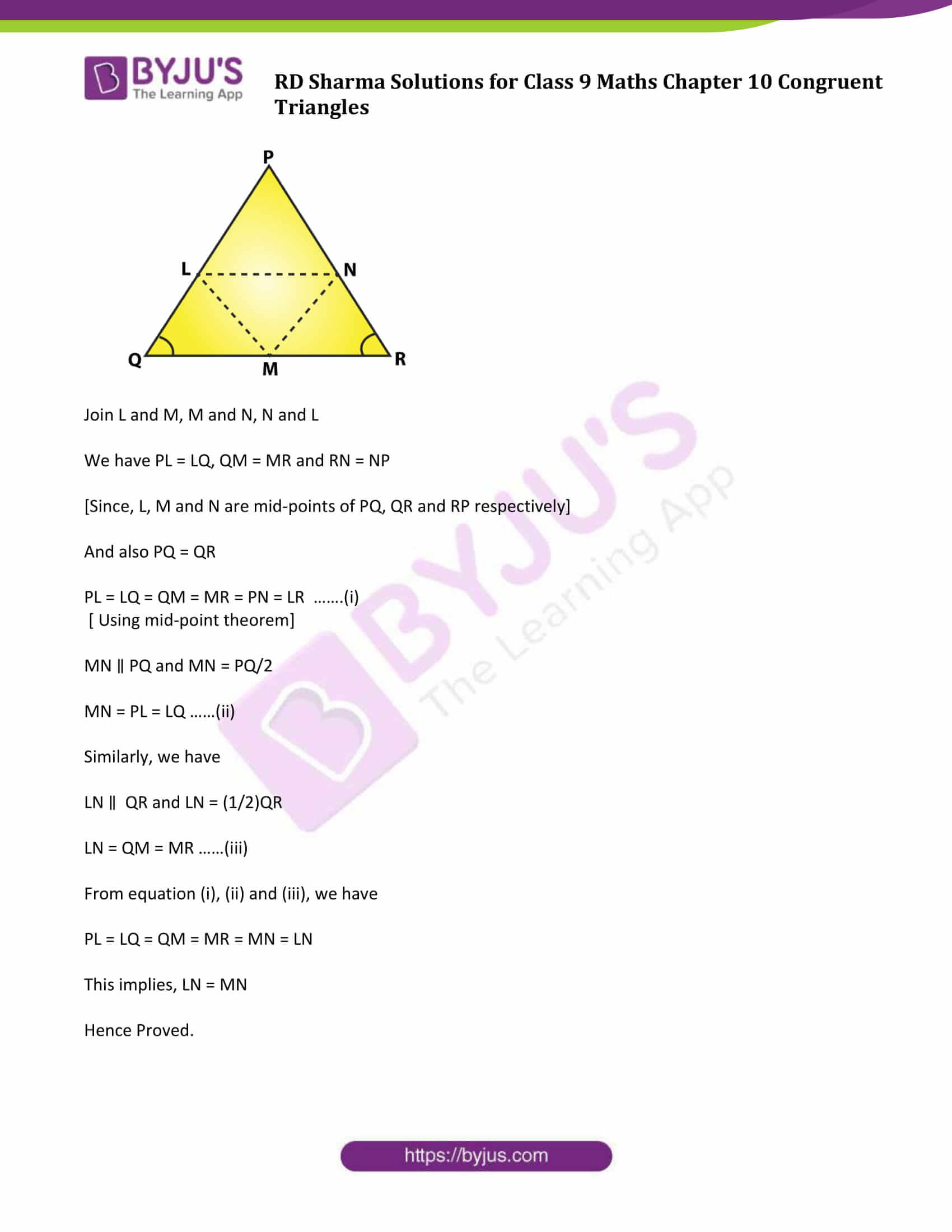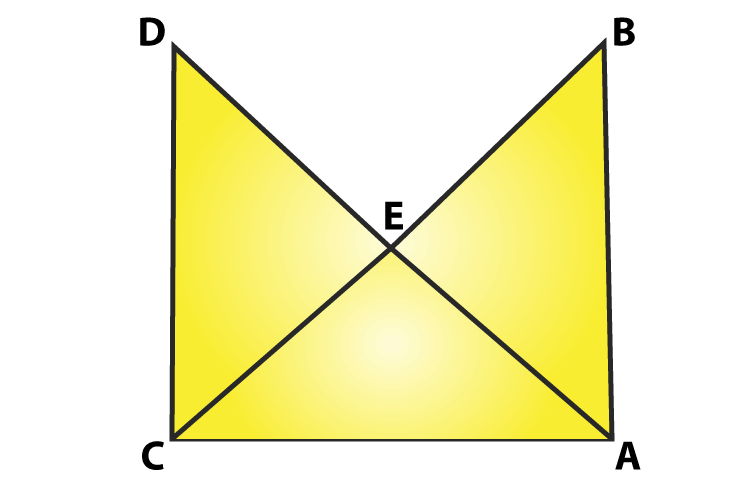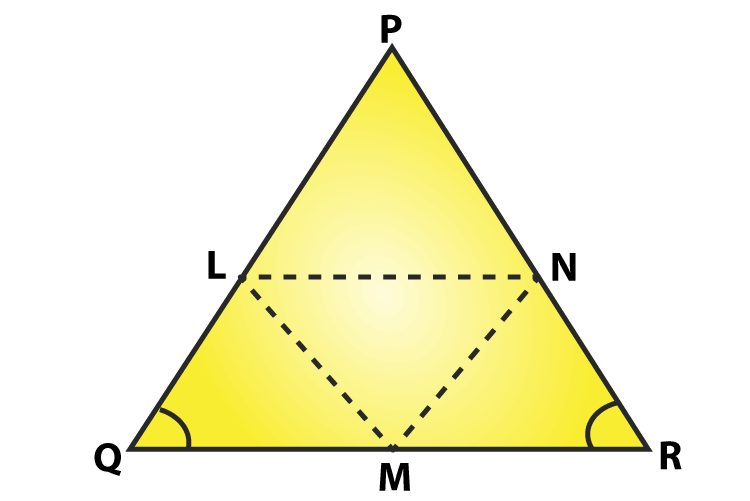# RD Sharma Solutions for Class 9 Maths Chapter 10 Congruent Triangles Exercise 10.4

RD Sharma class 9 Mathematics chapter 10 exercise 10.4 Congruent Triangles solutions are provided here. In this exercise, students will learn about congruence criterion of triangles mainly using SSS (Side-Side-Side). RD Sharma Class 9 Maths will also help students learn to solve problems and stating the criteria for the congruence of two triangles. Get the detailed RD Sharma solutions from the below link.

## Download PDF of RD Sharma Solutions for Class 9 Maths Chapter 10 Congruent Triangles Exercise 10.4### Access Answers to Maths RD Sharma Solutions for Class 9 Chapter 10 Congruent Triangles Exercise 10.4 Page number 10.47

Question 1: In figure, It is given that AB = CD and AD = BC. Prove that ΔADC ≅ ΔCBA.Solution:

From figure, AB = CD and AD = BC.

Consider Δ ADC and Δ CBA.

AB = CD [Given]

And AC = AC [Common side]

So, by SSS congruence criterion, we have

Hence proved.

Question 2: In a Δ PQR, if PQ = QR and L, M and N are the mid-points of the sides PQ, QR and RP respectively. Prove that LN = MN.

Solution:

Given: In Δ PQR, PQ = QR and L, M and N are the mid-points of the sides PQ, QR and RP respectively

To prove: LN = MNJoin L and M, M and N, N and L

We have PL = LQ, QM = MR and RN = NP

[Since, L, M and N are mid-points of PQ, QR and RP respectively]

And also PQ = QR

PL = LQ = QM = MR = PN = LR …….(i)

[ Using mid-point theorem]

MN ∥ PQ and MN = PQ/2

MN = PL = LQ ……(ii)

Similarly, we have

LN ∥ QR and LN = (1/2)QR

LN = QM = MR ……(iii)

From equation (i), (ii) and (iii), we have

PL = LQ = QM = MR = MN = LN

This implies, LN = MN

Hence Proved.

## RD Sharma Solutions for Class 9 Maths Chapter 10 Congruent Triangles Exercise 10.4

RD Sharma Solutions Class 9 Maths Chapter 10 Congruent Triangles Exercise 10.4 is based on the topic- Congruence Criterion – SSS (Side-Side-Side). According to the criteria, two triangles are congruent if the three sides of one triangle are equal to the corresponding three sides of the other triangle.